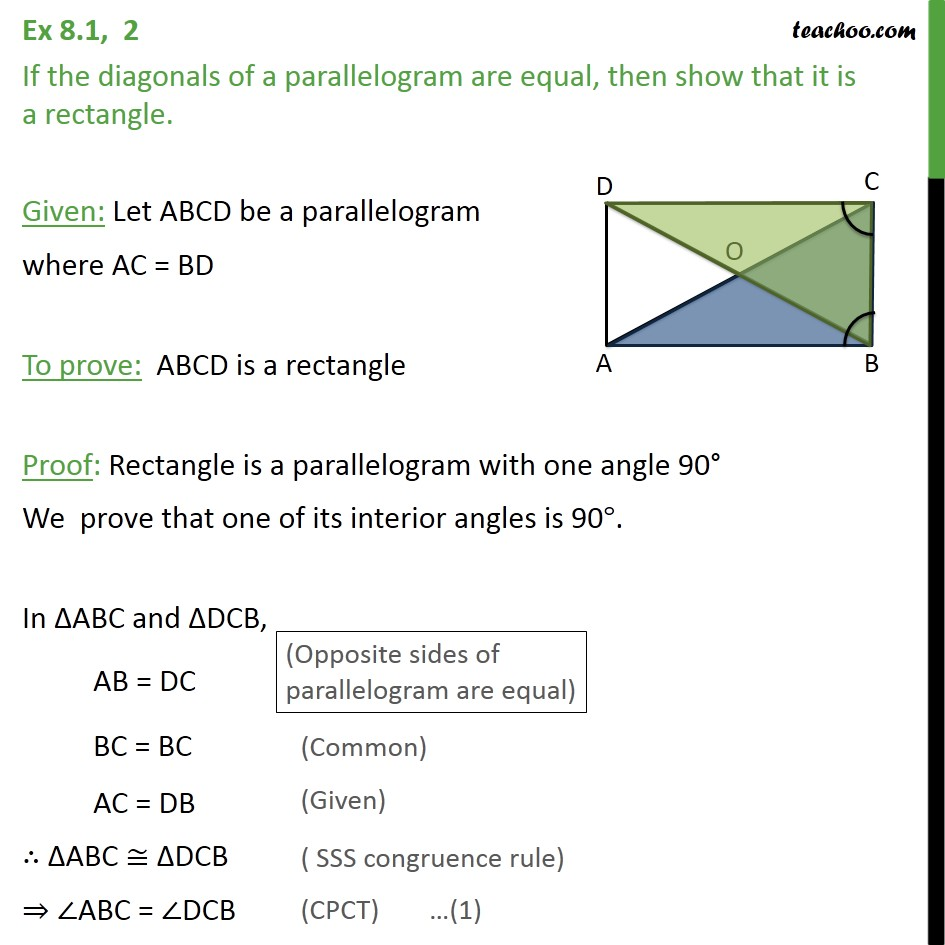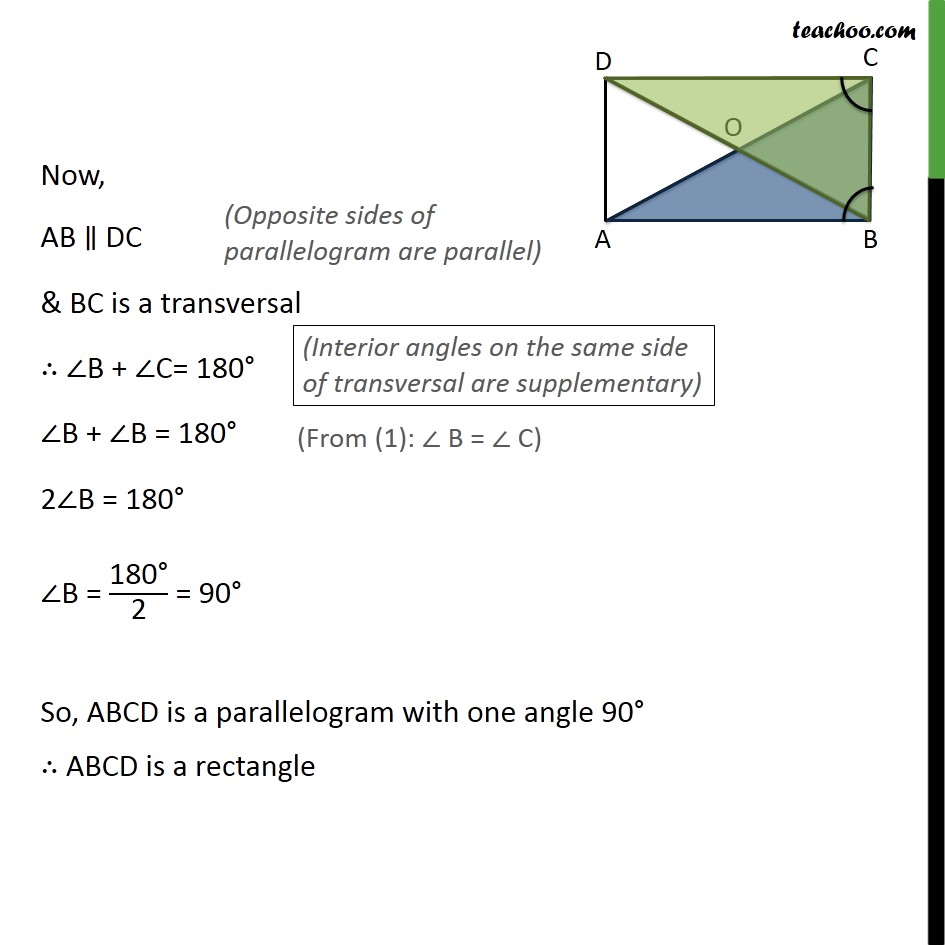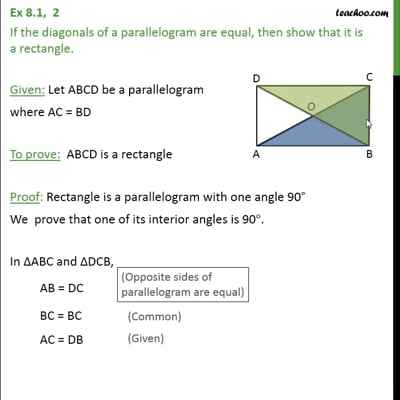Ex 8.1

Serial order wiseThis video is only available for Teachoo black users

Learn in your speed, with individual attention - Teachoo Maths 1-on-1 Class

### Transcript

Ex 8.1, 2 If the diagonals of a parallelogram are equal, then show that it is a rectangle. Given: Let ABCD be a parallelogram where AC = BD To prove: ABCD is a rectangle Proof: Rectangle is a parallelogram with one angle 90 We prove that one of its interior angles is 90 . In ABC and DCB, AB = DC BC = BC AC = DB ABC DCB ABC = DCB Now, AB DC & BC is a transversal B + C= 180 B + B = 180 2 B = 180 B = "180 " /"2" = 90 So, ABCD is a parallelogram with one angle 90 ABCD is a rectangle